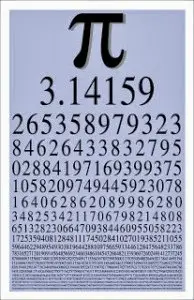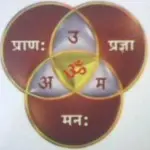# The Trivikram π - Pi The Secret of the Universe

In His discourses over the past few weeks, Parampoojya Bapu has been talking to us about "Om Ramvardayini Shreemahishasurmardinyai namah" of the 'Ankur-mantra' i.e. the second verse of the Shree Aniruddha Gurukshetram Mantra.In the course of these discourses Bapu acquainted us with the algorithms as also with the sacred symbols and representations of the Parameshvar. Bapu spoke to us in detail about the symbol of the Skanda (the Skanda-chinha), the Swastik, the Sun and the Moon in the Creation, the Deep (lamp), the Aarti and many more such algorithms.Later in the month of July, Bapu began His discourse saying, "We are going to talk about a great secret in the Universe." and then put us a question. "How did the mathematical constant Pi (п) come into being?" "Pi is a constant. It never changes, not even in the universe. We arrive at the constant Pi (п) by dividing the circumference with the diameter of a circle", He said. He then went on to explain the connection that Shree Hanumanta has with п (Pi) as also with the 'circle'. Quoting 'Brahmandabhovati vedhe vajrapuchchhe karu shakey'' (ब्रह्मांडाभोंवते वेढे वज्रपुच्छें करूं शके), a line from the Shree Maruti Stotra, He explained also, how the 'Brahmanda' (the cosmos) is at all times encircled by the 'puchchha' (the tail) of Shree Hanumanta. **The tip of Shree Hanumanta's tail, that is of 'vajra' is long enough to encircle (wind around) the whole of the cosmos.
In the discourse last week, i.e. on 8th August 2013, Bapu demonstrated to us the value of Pi (п) upto the 360th decimal. He grouped the figures (the decimals) in sets of five and explained why it was in sets of five that they were grouped.
Many harbour the misconception that 22/7 is indeed the exact value of Pi (п). But Pi(п) is actually a constant. 'Pi or п is an irrational number, which means that it cannot be expressed exactly as a ratio of any two integers. Fractions such as 22/7 are commonly used as an approximation of п; no fraction can be its exact value.' Mathematicians have till date succeeded in working out the value of Pi (п) up to the five millionth decimal place.Bapu said that in the Indian spiritual studies the concept of Pi (п) is represented by the Tripurari Trivikram symbol. Explaining it further He said, "The expanse of the cosmos (the Brahmaanda), i.e. of the universe created by the Aadimata is well-balanced. Shree Hanumanta signifies its limit and so He is the first son (Dattatreya, the sacred white light). The diameter of the cosmos is the Trivikram (Harihar) in other words Mahavishnu and Paramshiva together; Shreeram and Hanumant together; valour and forgiveness together.

The mathematical figure of 22/7 is but an approximation of Pi (п). It is the estimated value of Pi (п) and the world uses it thus in the field of mathematics.

Like Bapu told us, the Trivikram is infinite and so it is just impossible to work out the exact value of the mathematical expression of Pi (п).